# HSSlive: Plus One & Plus Two Notes & Solutions for Kerala State Board

## AP Board Class 7 Maths Chapter 9 Construction of Triangles Ex 1 Textbook Solutions PDF: Download Andhra Pradesh Board STD 7th Maths Chapter 9 Construction of Triangles Ex 1 Book AnswersAP Board Class 7 Maths Chapter 9 Construction of Triangles Ex 1 Textbook Solutions PDF: Download Andhra Pradesh Board STD 7th Maths Chapter 9 Construction of Triangles Ex 1 Book Answers

## Andhra Pradesh State Board Class 7th Maths Chapter 9 Construction of Triangles Ex 1 Books Solutions

 Board AP Board Materials Textbook Solutions/Guide Format DOC/PDF Class 7th Subject Maths Chapters Maths Chapter 9 Construction of Triangles Ex 1 Provider Hsslive

2. Click on the Andhra Pradesh Board Class 7th Maths Chapter 9 Construction of Triangles Ex 1 Answers.
3. Look for your Andhra Pradesh Board STD 7th Maths Chapter 9 Construction of Triangles Ex 1 Textbooks PDF.
4. Now download or read the Andhra Pradesh Board Class 7th Maths Chapter 9 Construction of Triangles Ex 1 Textbook Solutions for PDF Free.

## AP Board Class 7th Maths Chapter 9 Construction of Triangles Ex 1 Textbooks Solutions with Answer PDF Download

Find below the list of all AP Board Class 7th Maths Chapter 9 Construction of Triangles Ex 1 Textbook Solutions for PDF’s for you to download and prepare for the upcoming exams:

Question 1.
Construct ∆ ABC in which AB = 5.5 cm, BC = 6.5 cm and CA = 7.5 cm.
Solution:
AB = 5.5 cm, BC = 6.5 cm, CA = 7.5 cm.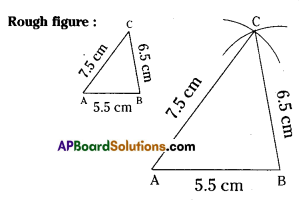Step – 1: Draw a rough sketch of the triangle and label it with the given measurements.
Step – 2: Draw a line segment AB of A length 5.5 cm.
Step – 3: With A as centre, draw an arc of radius 7.5 cm.
Step – 4: With B as centre, draw another arc of radius 6.5 cm such that it intersects first arc at C.
Step – 5: Join A, C and B, C. The required ABC is constructed.

Question 2.
Construct ∆ NIB in which NI = 5.6 cm, IB = 6 cm and BN = 6 cm. What type of triangle is this?
Solution:
NI = 5.6 cm, IB = 6 cm, BN = 6 cm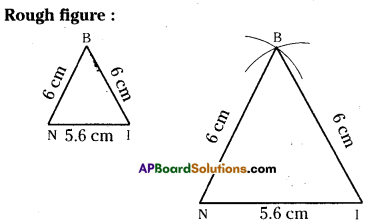Step – 1: Draw a rough sketch of the trIangle and label It with the given measurements.
Step – 2: Draw a line segment NI of length 5.6 cm. ,
Step – 3: With N as centre, draw an arc of radius 6 cm.
Step – 4: With I as centre, draw another arc of radius 6 cm such that it intersects first arc at B.
Step – 5: JoIn N, B and I, B. The required triangle ∆ NIB is constructed.
This triangle ¡s an Isosceles triangle.

Question 3.
Construct an equilateral ∆ APE with side 6.5 cm.
Solution: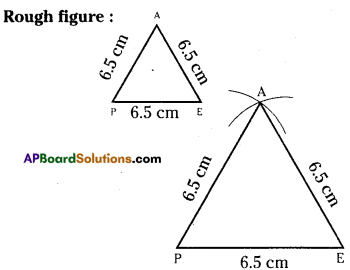AP = FE = EA : 6.5 cm.
Step – 1: Draw a rough sketch of the triangle and label it with the given measurements.
Step – 2: Draw a line segment PE of length 6.5 cm.
Step – 3: With P as centre, draw an arc of radius 6.5 cm.
Step – 4: WIth E as centre, draw another arc of radius 6.5 cm such that it intersects first arc at A.
Step – 5: Join P, A and E, A. The required ∆AFE is constructed.

Question 4.
Construct a ∆ XYZ in which XY = 6 cm, YZ = 8 cm and ZX = 10 cm. Using protractor find the angle at X. What type of triangle is this?
Solution:
XY = 6cm, YZ = 8cm, ZX = 10cm.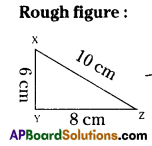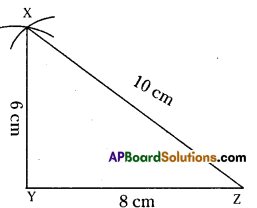Step – 1: Draw a rough sketch of the triananle and label it with the given measurements.
Step – 2: Draw a line segment YZ of length of 8 cm.
Step – 3: With Y as centre, draw an arc of radius 6 cm.
Step – 4: With Z as centre, draw another arc of radius 10 cm such that it intersects first arc at X.
Step – 5: Join Y, X and Z, X. The required XYZ is constructed ∠X = 52°
This triangle is an acute angled triangle.

Question 5.
Construct ∆ ABC in which AB =4 cm, BC = 7 cm and CA= 3 cm. Which type of triangle is this?
Solution:
AB = 4 cm, BC = 7 cm, CA = 3 cm.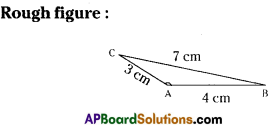Step – 1: Draw a rough sketch of the triangle and label it with the given measurements.
Step – 2: Draw a line segment AB of length 4 cm.
Step – 3: With A as centre, draw an arc of radius 3 cm.
Step – 4: With B as centre, draw another arc of radius 7 cm such that it intersects first arc at C.
Step – 5: Join C, A and C, B. The required ∆ABC is constructed.
This triangle Is an obtuse angled triangle.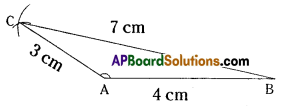Question 6.
Construct ∆ PEN with PE = 4 cm, EN =5 cm and NP =3 cm. If you draw circles instead of arcs how many points of intersection do you get? How many triangles with given measurements are possible? Is this true in case of every triangle?
Solution:PE = 4cm,EN = 5cm, NP = 3crn.
Step – 1: Draw a rough sketch of the triangle and label it with the given measurements.
Step – 2: Draw a line segment EN of length 5 cm.
Step – 3: With E as centre, draw on arc (circle) of radius 4 cm.
Step – 4: With N as centre, draw another arc (circle) of radius 3 cm such that it intersects first arc at P.
Step – 5: Join E, P and N, P. The required ∆PEN is constructed,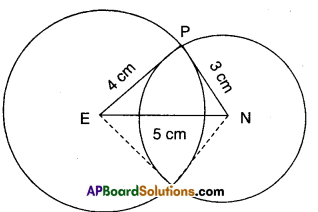if we draw circles instead of arcs, we get two points of intersection. Then, we can draw two triangles with the given measurements.
Yes, this is true in case of every triangle.

## Andhra Pradesh Board Class 7th Maths Chapter 9 Construction of Triangles Ex 1 Textbooks for Exam Preparations

Andhra Pradesh Board Class 7th Maths Chapter 9 Construction of Triangles Ex 1 Textbook Solutions can be of great help in your Andhra Pradesh Board Class 7th Maths Chapter 9 Construction of Triangles Ex 1 exam preparation. The AP Board STD 7th Maths Chapter 9 Construction of Triangles Ex 1 Textbooks study material, used with the English medium textbooks, can help you complete the entire Class 7th Maths Chapter 9 Construction of Triangles Ex 1 Books State Board syllabus with maximum efficiency.

## FAQs Regarding Andhra Pradesh Board Class 7th Maths Chapter 9 Construction of Triangles Ex 1 Textbook Solutions

#### Can we get a Andhra Pradesh State Board Book PDF for all Classes?

Yes you can get Andhra Pradesh Board Text Book PDF for all classes using the links provided in the above article.

## Important Terms

Andhra Pradesh Board Class 7th Maths Chapter 9 Construction of Triangles Ex 1, AP Board Class 7th Maths Chapter 9 Construction of Triangles Ex 1 Textbooks, Andhra Pradesh State Board Class 7th Maths Chapter 9 Construction of Triangles Ex 1, Andhra Pradesh State Board Class 7th Maths Chapter 9 Construction of Triangles Ex 1 Textbook solutions, AP Board Class 7th Maths Chapter 9 Construction of Triangles Ex 1 Textbooks Solutions, Andhra Pradesh Board STD 7th Maths Chapter 9 Construction of Triangles Ex 1, AP Board STD 7th Maths Chapter 9 Construction of Triangles Ex 1 Textbooks, Andhra Pradesh State Board STD 7th Maths Chapter 9 Construction of Triangles Ex 1, Andhra Pradesh State Board STD 7th Maths Chapter 9 Construction of Triangles Ex 1 Textbook solutions, AP Board STD 7th Maths Chapter 9 Construction of Triangles Ex 1 Textbooks Solutions,
Share: